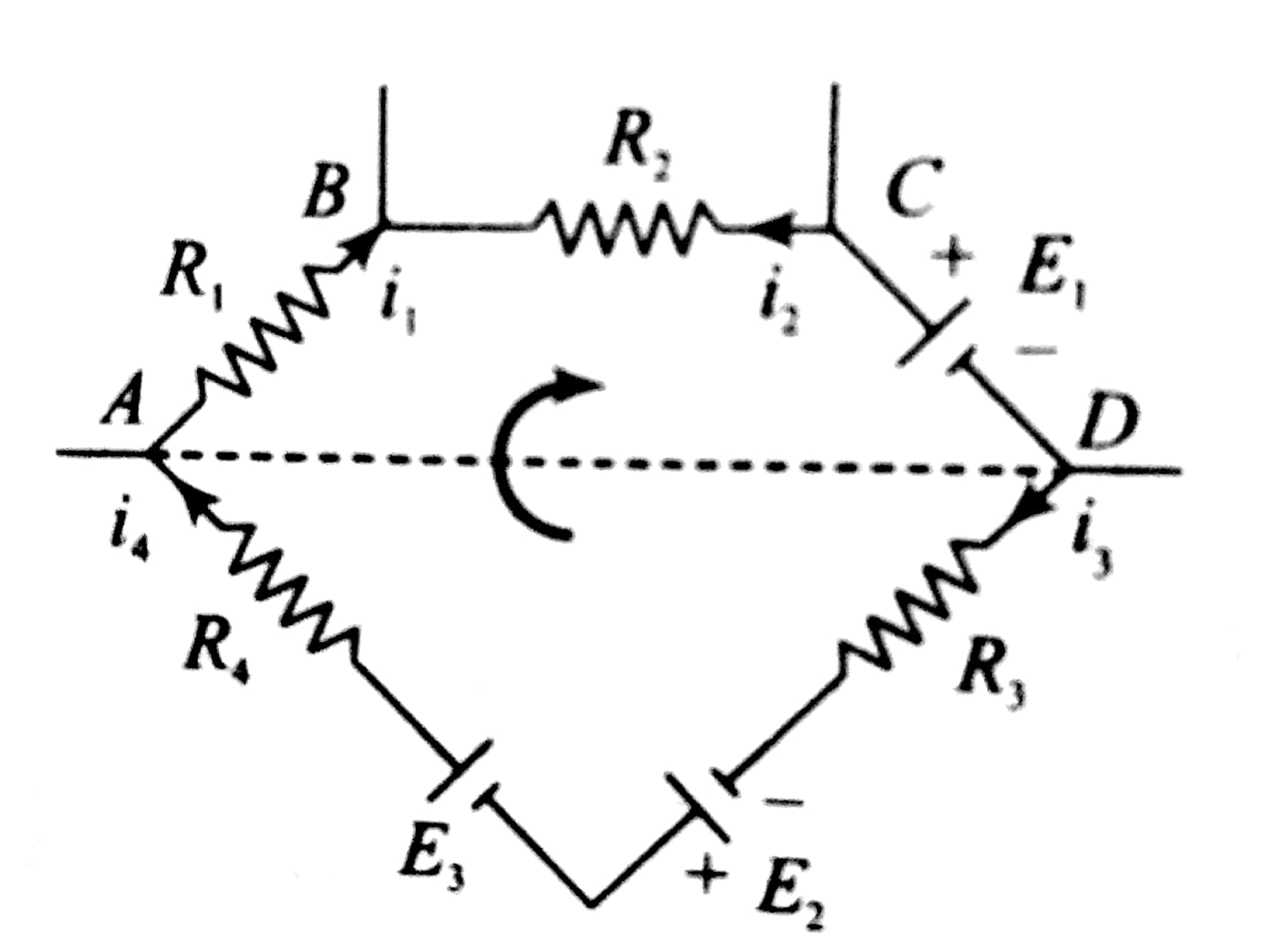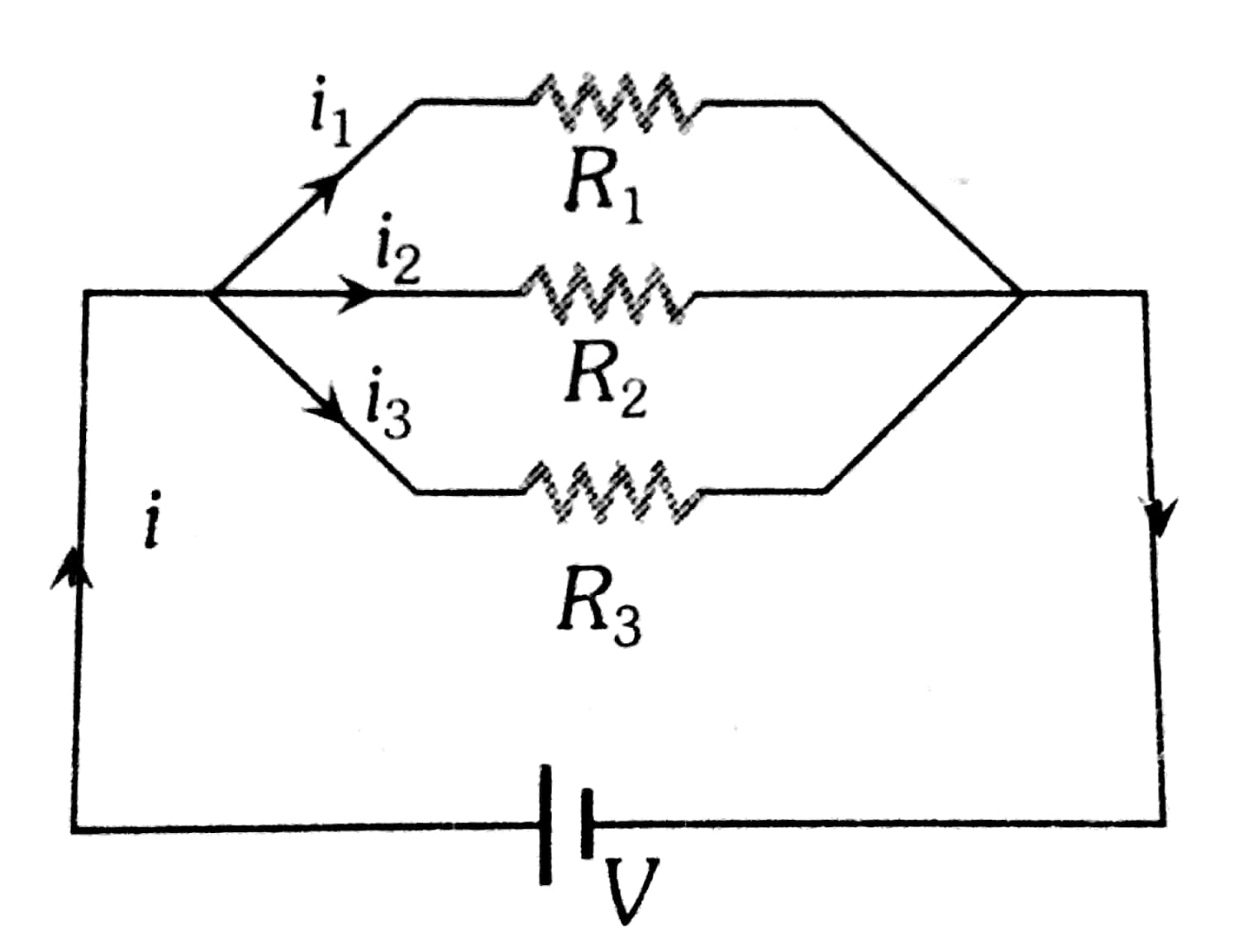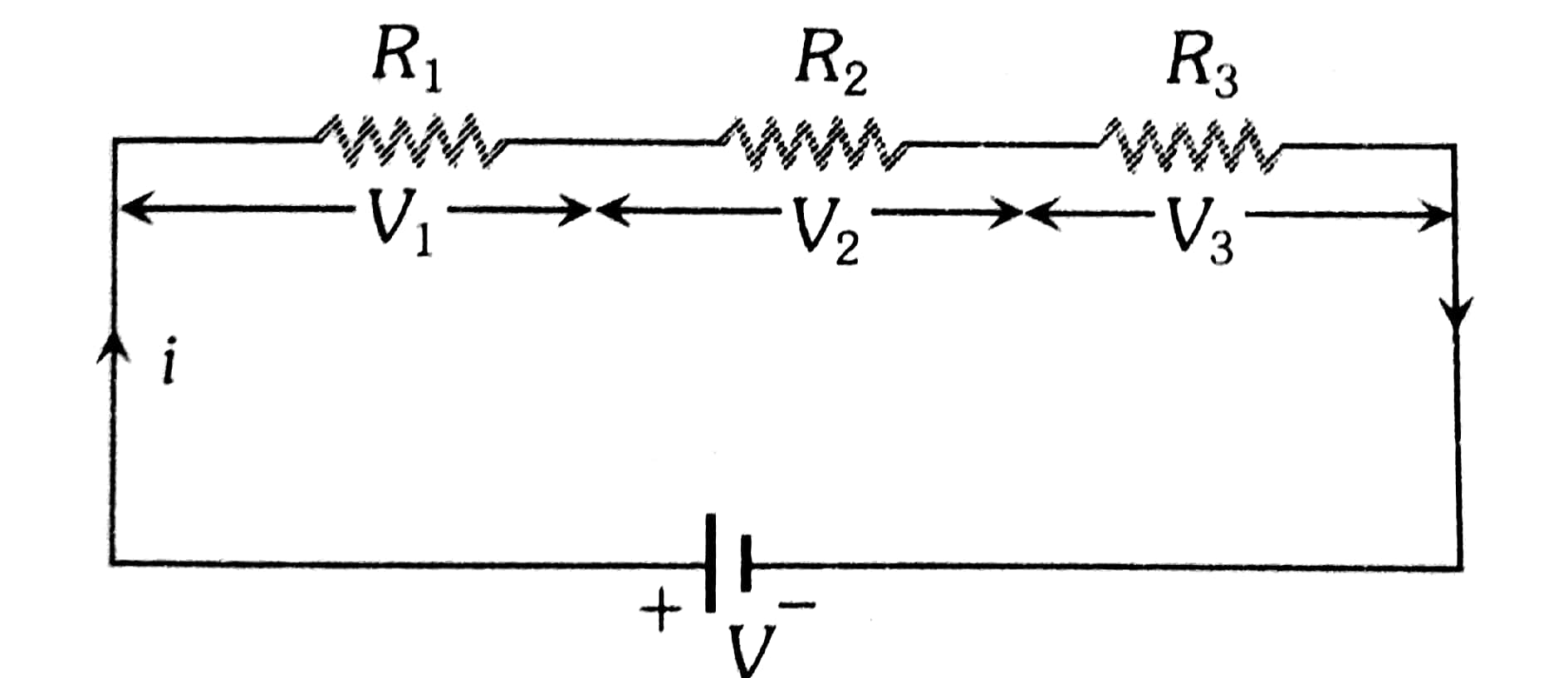## Filters

Sort by :
Q

Letandbe the roots of equationis equal to:

• Option 1)

6

• Option 2)

-6

• Option 3)

3

• Option 4)

-3

3

Engineering
30 Views   |

LetwhereThenequals :

• Option 1)• Option 2)• Option 3)• Option 4)Option 2

Engineering
53 Views   |

Let ABC be a triangle whose circumcentre is at P.  If the position vectors of A, B, C

and P arerespectively, then the position vector of the orthocentre of this triangle, is :

• Option 1)• Option 2)• Option 3)• Option 4)Engineering
56 Views   |

If (10)9 + 2(11)1   (10)8 + 3(11)2  (10)7 +......  +10 (11)9 = k (10)9, then k is equal to :

• Option 1)

100

• Option 2)

110

• Option 3)• Option 4)Use

Sum of n terms of a GP -

- wherein

first term

common ratio

number of terms

and

(10)9 + 2(11)1 (10)8 + 3(11)2 (10)7 +......  +10 (11)9 = k(10)9

Take common 109

Subtract

Option 1)

100

Option 2)

110

Option 3)Option 4)View More
Engineering
64 Views   |

In a large building, there are 15 bulbs of   40 W, 5 bulbs of 100 W, 5 fans of 80 W and 1 heater of 1 kW.  The voltage of the electric mains is 220 V.  The minimum capacity of the main fuse of the building will be :

• Option 1)

8 A

• Option 2)

10 A

• Option 3)

12 A

• Option 4)

14 A

As we have learned

In parallel Grouping -

-

Resistance of each bulb of 40 W

Resistance of 15 bulbs = 1210/15 = 242/3

Resistance of 100 W bulb =

Resistance of 5 such bulbs = 485/5

Resistance of fan =     =  605

Resistance of 5 such fan  = 605 /5

= 121

Resistance of heater =

Equivalent resistance

R = 484 / 25

Option 1)

8 A

Option 2)

10 A

Option 3)

12 A

Option 4)

14 A

View More
Engineering
43 Views   |

In the circuit shown, current (in A) through the 50 V and 30 V batteries are, respectively :• Option 1)

2.5 and 3

• Option 2)

3.5 and 2

• Option 3)

4.5 and 1

• Option 4)

3  and 2.5

As we discussed in

Cell -

The device which converts Chemical energy into electrical energy is known as electric cell.

Option 1)

2.5 and 3

Option 2)

3.5 and 2

Option 3)

4.5 and 1

Option 4)

3  and 2.5

Engineering
45 Views   |

A d.c. main supply of e.m.f. 220 V is connected across a storage battery of e.m.f. 200 V through a resistance of 1. The battery terminals are connected to an external resistance ‘R’. The minimum value of ‘R’, so that a current passes through the battery to charge it is :

• Option 1)• Option 2)• Option 3)• Option 4)

zero

Option 1)Option 2)Option 3)Option 4)

zero

View More
Engineering
111 Views   |In the circuit shown, the current in the 1resistor is :

• Option 1)

1.3 A ,from P to Q

• Option 2)

0 A

• Option 3)

0.13 A ,from Q to P

• Option 4)

0.13 A ,from P to Q

answer is option 3)0.13A from Q  to P

•  Let, Current i1 from 6v battery passes through 3 ohm resistance . At Q current breaks and current i2 goes through 3 ohm and ( i1-i2 ) goes through 1 ohm ,.
• Applying, kirchoffs law in 1st and 2nd loop,we get two eqns as follows
• 4i1-i2=6
• 6i2-i1=9
• solving ,,  i1-i2 =0.13 A
View More
Engineering
83 Views   |

In the electric network shown, when no current flows through theresistor in the arm EB, the potential difference between the points A and D will be :• Option 1)

3V

• Option 2)

4V

• Option 3)

5V

• Option 4)

6V

The potential difference can be calculated via following method:

View More
Engineering
74 Views   |

The supply voltage to a room is 120 V. The resistance of the lead wires is 6. A 60 W bulb is already switched on. What is the decrease of voltage across the bulb, when a 240 W heater is switched on in parallel to the bulb?

• Option 1)

10.04 Volt

• Option 2)

Zero Volt

• Option 3)

2.9 Volt

• Option 4)

13.3 Volt

Need details on this question

Engineering
77 Views   |

Ifandbe the threshold wavelength and wavelength of incident light, the velocity of photoelectron ejected from the metal surface is :

• Option 1)• Option 2)• Option 3)• Option 4)As discussed in the concept

Photoelectric Effect -

- wherein

where

m is the mass of the electron

u is the velocity associated with the ejected electron.

h is plank’s constant.

v is frequency of photon,

v0 is threshold frequency of metal.

Hence the correct option is 3

Option 1)Option 2)Option 3)Option 4)View More
Engineering
72 Views   |

Chloro compound of Vanadium has only spin magnetic moment of 1.73 BM.  This Vanadium chloride has the formula :

(at. no. of V=23)

• Option 1)

VCl2

• Option 2)

VCl4

• Option 3)

VCl3

• Option 4)

VCl5

As discussed in

Magnetic Quantum Number (m) -

It  gives information about the spatial orientation of the orbital with respect to standard set of co-ordinate axis.

-

The value of magnetic moment is 1.73 BM

where n is the number of unpaired electrons

After calculation n = 1

To obtain one unpaired electron V should be tetrapositive ion and the formula of its chlorid should be

Option 2 is correct

Option 1)

VCl2

Incorrect option

Option 2)

VCl4

Correct option

Option 3)

VCl3

Incorrect option

Option 4)

VCl5

Incorrect option

View More
Engineering
63 Views   |

Resistance of a given wire is obtained  by measuring the current flowing in it and the voltage difference applied across it. If the percentage errors in the measurement of the current and the voltage difference are 3% each, then error in the value of resistance of the wire is

• Option 1)

6%

• Option 2)

zero

• Option 3)

1%

• Option 4)

3%

As discussed in

Ohm's Law -

Current flowing through the conductor is directly proportional to the Potential difference accross two ends .

- wherein

unit -

Electric Resistance

Percentage error in is

Option 1)

6%

This option is correct.

Option 2)

zero

This option is incorrect.

Option 3)

1%

This option is incorrect.

Option 4)

3%

This option is incorrect.

View More
Engineering
65 Views   |

This question has Statement I and Statement II. Of the four choices given after the Statements, choose the one that best describes the two Statemens.

Statement - I : Higher the range, greater is the resistance of ammeter.

Statement - II : To increase the range of ammeter,additional shunt needs to be used across it.

• Option 1)

Statement - I is false, Statement - II is true.

• Option 2)

Statement - I is true, Statement - II is true, Statement - II is the correct explanation of Statement - I.

• Option 3)

Statement - I is true, Statement - II is true, Statement - II is not the correct explanation of Statement - I.

• Option 4)

Statement - I is true, Statement - II is false.

As discussed in-

Required shunt -

- wherein

Current through galvanometer

The answer follows from basic Principle of stunt and its use to make an ammeter:

Option 1)

Statement - I is false, Statement - II is true.

This option is correct.

Option 2)

Statement - I is true, Statement - II is true, Statement - II is the correct explanation of Statement - I.

This option is incorrect.

Option 3)

Statement - I is true, Statement - II is true, Statement - II is not the correct explanation of Statement - I.

This option is incorrect.

Option 4)

Statement - I is true, Statement - II is false.

This option is incorrect.

View More
Engineering
77 Views   |

A 10 V battery with internal resistanceand a 15V battery with internal resistanceare connected in parallel to a voltmeter (see figure). The reading in the voltmeter will be close to :• Option 1)

11.9 V

• Option 2)

12.5 V

• Option 3)

13.1 V

• Option 4)

24.5 V

As we discussed in

In closed loop -

- whereinCurrent in the circuit

Option 1)

11.9 V

The option is incorrect

Option 2)

12.5 V

The option is incorrect

Option 3)

13.1 V

The option is correct

Option 4)

24.5 V

The option is incorrect

View More
Engineering
27 Views   |

A 3 olt battery with  negligible internal resistance is connected in a circuit as shown in the figure. The current I i n the circuit will be• Option 1)• Option 2)• Option 3)• Option 4)as discussed in

Parallel Grouping -

Potential - Same

Current - Different

- whereinOption 1)This option is incorrect.

Option 2)This option is correct.

Option 3)This option is incorrect.

Option 4)This option is incorrect.

View More
Engineering
32 Views   |

Two electric bulbs marked  25 W-220 V and 100 W - 220 V are connected in series to a 440 V supply .Which of the bulbs will fuse?

• Option 1)

both

• Option 2)

100W

• Option 3)

25 W

• Option 4)

neither

As discussed in:

Series Grouping -

Potential - Different

Current - Same

- whereinSimilarly,

The current flowing through the circuit

After solving this, we get

Thus the bulb rated 25W-220V will fuse.

Option 1)

both

Option 2)

100W

Option 3)

25 W

Option 4)

neither

View More
Engineering
176 Views   |

Three capacitances, each of 3F, are  provided. These cannot be combined to provide the resultant capacitance of :

• Option 1)• Option 2)• Option 3)• Option 4)3

Engineering
96 Views   |

The magnitude of the average electric field normally present in the atmosphere just above the surface of the Earth is about

150 N/C, directed inward towards the center of the Earth.  This gives the total net surface charge carried by the Earth to  be:• Option 1)

+670 kC

• Option 2)

- 670 kC

• Option 3)

- 680 kC

• Option 4)

+ 680 kC

As we discussed in the concept

Infinite Plane parallel sheets of charge -

If

and

and

-

Electric Field E = 150 N/C

Total surface charge carried by earth q= ?

As electric field is directed inwards, hence,

Option 1)

+670 kC

Option 2)

- 670 kC

Option 3)

- 680 kC

Option 4)

+ 680 kC

View More
Engineering
194 Views   |

In the given circuit diagram when the current reaches steady state in the circuit, the charge on the capacitor of capacitance C will be :• Option 1)• Option 2)• Option 3)• Option 4)As we discussed in concept

# Charging Of Capacitors

where in

at steady state there is no current through r1

Q=Option 1)Option 2)Option 3)Option 4)View More
Exams
Articles
Questions